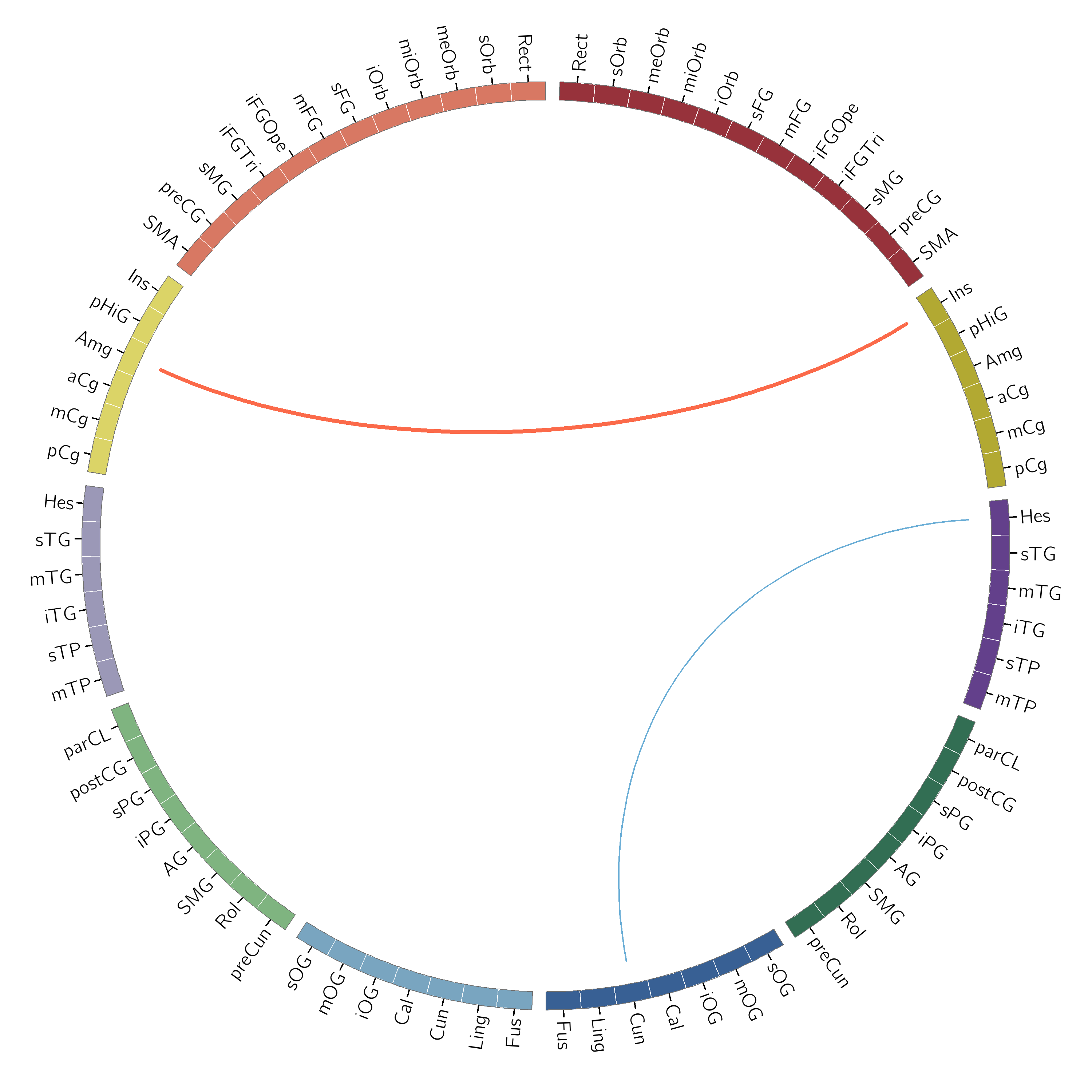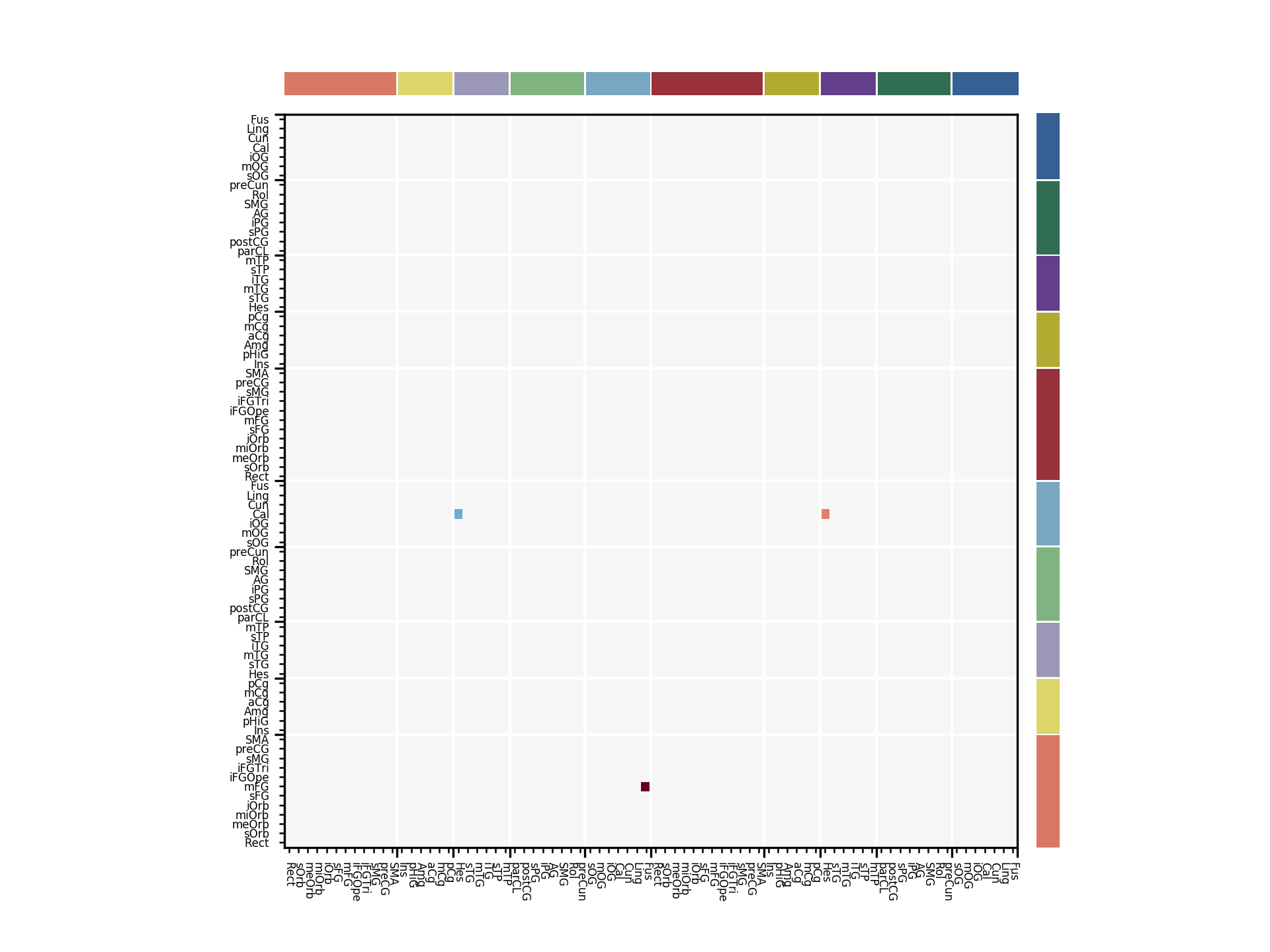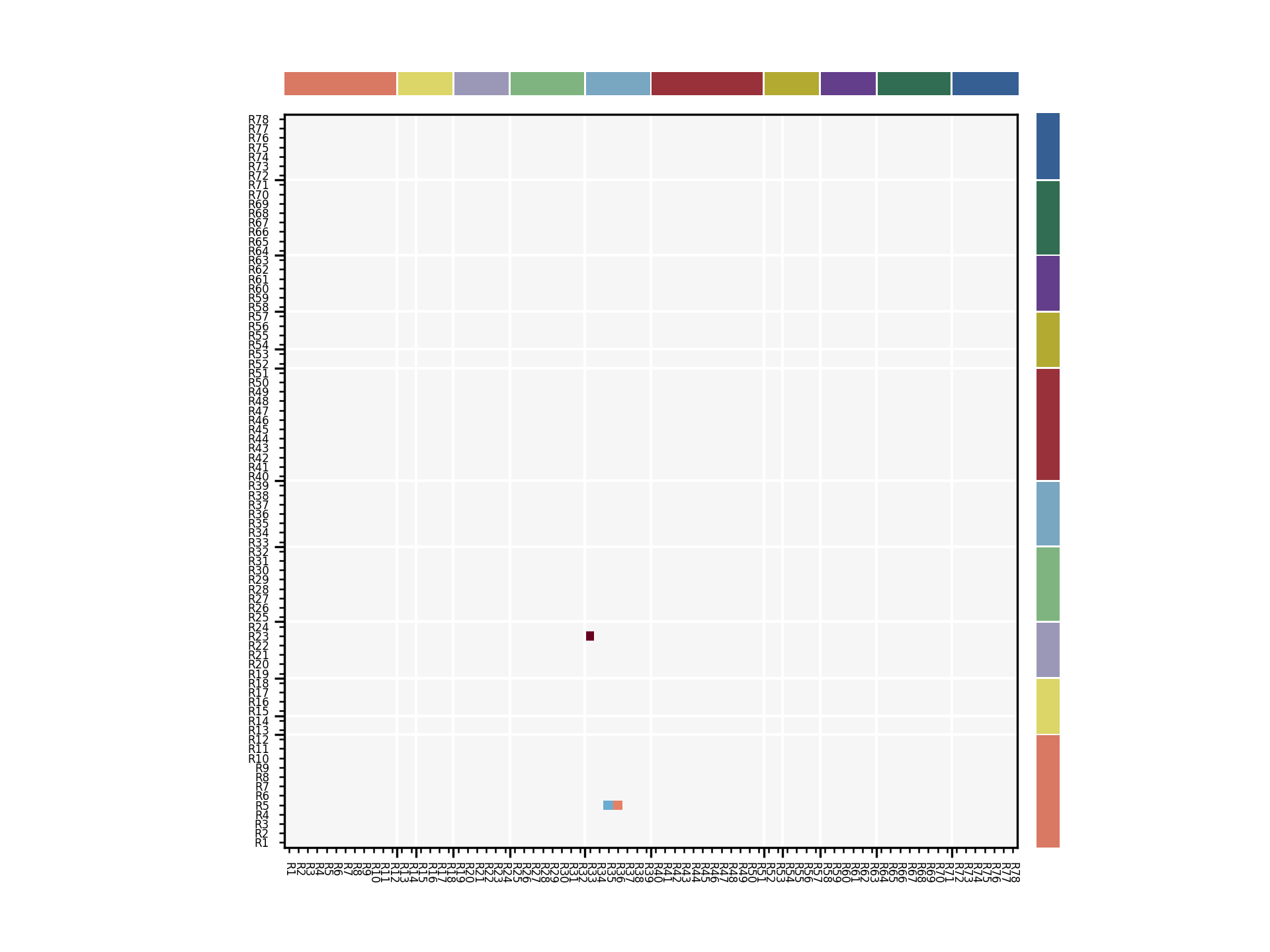# Tutorial 7 - Plotting Helpers¶

In this tutorial, we will explore the plotting helper functions which are available for use in sails; primarily for plotting netmats and circular connectivity pots.

For this tutorial, we will use some example plot definition files which are provided with sails.

We start by importing our modules and finding and finding our example path

from os.path import join

from sails import find_example_path

ex_dir = find_example_path()

group_csv = join(ex_dir, 'aal_cortical_plotting_groups.csv')
region_csv = join(ex_dir, 'aal_cortical_plotting_regions.csv')


The two files which are imported above describe the layout of the plots which we will create. The example files given are for a specific cortical subset of the AAL atlas which we have used in some of our own work; this parcellation contains 78 regions; 39 per hemisphere.

The groups.csv file contains a list of the groups of regions which we will plot. In this case, we have divided the 78 regions into 10 groups:

• Frontal left / right
• Medial left / right
• Temporal left / right
• Parietal left / right
• Occipital left / right

The regions.csv file describes each ROI and places it within these groups. It also provides the indexing information so that we can match between our netmats and the plots.

More details of both of these files can be found in the docstring for the from_csv_files function of the sails.CircosHandler class.

We will now build a connectivity diagram using this structure.

## Setting up the handler¶

We start by setting up a handler which parses the CSV files:

from sails import CircosHandler

c = CircosHandler.from_csv_files(group_csv, region_csv)


There is one additional argument available to the from_csv_files function; this argument is analysis_column. By default, the order in which our analysed data is stored comes from the AnalysisID column of the regions.csv spreadsheet. You can alter which column is used for this by setting the analysis_column argument to the function. This is useful in cases where you have data in different orders from different analyses but where you want to produce plots with the same ordering with little effort.

## Extracting Region Ordering and Indices¶

We can use use this handler to extract various orderings of our ROIs and the indices needed to re-order data into them.

# Show the order in circular form (clockwise) of the groups
print(c.circular_groups)

['frontal_L', 'medial_L', 'temporal_L', 'parietal_L', 'occipital_L', 'occipital_R', 'parietal_R', 'temporal_R', 'medial_R', 'frontal_R']

# Show the order in netmat form (top to bottom) of the groups
print(c.netmat_groups)

['frontal_L', 'medial_L', 'temporal_L', 'parietal_L', 'occipital_L', 'frontal_R', 'medial_R', 'temporal_R', 'parietal_R', 'occipital_R']

# Show the last 10 regions in circular order and the corresponding
# indices into our data
print(c.circular_regions[-10:])
print(c.circular_indices[-10:])

['Frontal_Sup_Medial_R', 'Frontal_Inf_Tri_R', 'Frontal_Inf_Oper_R', 'Frontal_Mid_R', 'Frontal_Sup_R', 'Frontal_Inf_Orb_R', 'Frontal_Mid_Orb_R', 'Frontal_Med_Orb_R', 'Frontal_Sup_Orb_R', 'Rectus_R']
[28, 19, 15, 25, 31, 17, 24, 21, 30, 61]

# Show the last 10 regions in netmat order and the corresponding
# indices into our data
print(c.netmat_regions[-10:])
print(c.netmat_indices[-10:])

['SupraMarginal_R', 'Rolandic_Oper_R', 'Precuneus_R', 'Occipital_Sup_R', 'Occipital_Mid_R', 'Occipital_Inf_R', 'Calcarine_R', 'Cuneus_R', 'Lingual_R', 'Fusiform_R']
[67, 63, 59, 45, 43, 41, 5, 13, 39, 33]


## Circular Plots¶

To generate circular plots, we use the program circos. You will need to have the program installed on your computer in order to generate such plots. circos is available from http://circos.ca/software/. On Debian and similar, you can simply apt install circos circos-data libsvg-perl.

Note that if you are creating plots for publication using Circos, you should cite the relevant publication: Krzywinski, M. et al. Circos: an Information Aesthetic for Comparative Genomics. Genome Res (2009) 19:1639-1645.

### Generating a karyotype¶

Circos uses the term karyotype to describe the ordering of chromosomes and bands within them. In our case, we are using this to describe groups of regions and individual ROIs respectively. We only need to generate a karyotype file once for each layout; we do not need a different karyotype file for each individual plot.

To generate a karytotype file, we open a file and write the contents of the karyotype() function into it.

f = open('aal_karyotype.txt', 'w')
f.write(c.karyotype())
f.close()


In our example case, the start of the file will look like this:

chr - frontal_L 1 0 12000 red
chr - medial_L 2 0 6000 yellow
chr - temporal_L 3 0 6000 purple
chr - parietal_L 4 0 8000 green
chr - occipital_L 5 0 7000 blue
chr - occipital_R 6 0 7000 vvdblue
chr - parietal_R 7 0 8000 vvdgreen
chr - temporal_R 8 0 6000 vvdpurple
chr - medial_R 9 0 6000 vvdyellow
chr - frontal_R 10 0 12000 vvdred
band frontal_L Rectus_L Rectus_L 0 1000 grey
band frontal_L Frontal_Sup_Orb_L Frontal_Sup_Orb_L 1000 2000 grey


We will use this file in the next section to make our plots.

### Generating a connectivity plot based on the karyotype¶

We start by generating some controlled data with connections between only a few regions

import numpy as np

data = np.zeros((78, 78)) * np.nan

# Add a strong positive connection between Amygdala_L and Insula_R
data[0, 37] = 10.0
# Add a weaker negative connection between Cuneus_R and Heschl_R
data[13, 35] = -4.0


Note that when the data values are used in the circular plotting routines, they will be used as line widths in pixels.

We now generate a set of circos configuration files from this data. We also need to pass it our karyotype file name and the output base name.

c.gen_circos_files(data, 'aal_karyotype.txt', 'aal_test_out')


The code above assumes that you have the circos config files installed in /etc/circos. If you have them in another location, pass the circos_path variable to the gen_circos_files routine, e.g.:

c.gen_circos_files(data, 'aal_karyotype.txt', 'aal_test_out',
circos_path='/usr/local/etc/circos')


This will generate two files: aal_test_out.conf and aal_test_out.txt. The former is the circos configuration file and the latter is the file which contains the actual information about the connections. In our case, we can see that the latter only contains two lines; one for each of our two connections.

### Manually Generating the plot¶

To manually generate the plot from the configuration files, we use a normal shell and run the circos command.

circos -conf aal_test_out.conf


You may get an error which contains the following:

*** CIRCOS ERROR ***

cwd: /tmp

command: /usr/bin/circos -conf aal_test_out.conf

CONFIGURATION FILE ERROR


This is because of a problem with circos finding some of its configuration files. You can fix this by running the following commands from your shell (whilst in the directory containing the files). This assumes that the circos config files are in /etc/circos:

mkdir etc
ln -s /etc/circos/tracks etc/


You should then re-run the circos command.

Two files will be created: test_out.png and test_out.svg. The image should look like this:### Automatically Generating the plot¶

Instead of going to the effort of the above, there is a helper routine gen_circos_plot which will create a temporary directory, generate all of the configuration files, run circos and then copy the plots to your final destination. It can be used directly in place of gen_circos_files. If the circos call fails, an exception will be raised which will include the contents of stdout and stderr so that you can investigate the problem.

Note that you do not pass a karyotype file name to the gen_circos_plot routine as it will generate a karyotype file in the temporary directory for you.

c.gen_circos_plot(data, 'aal_test_out',
circos_path='/usr/local/etc/circos')


## Netmat Plots¶

In this section, we will discuss how to use the netmat plotting routines which come as part of the CircosHandler above. Further down there is documentation on how to use the raw plot_netmat routine.

We assume that we are in the same session as above and that c still represents a CircosHandler object.

# Set up some netmat data
netdata = np.zeros((78, 78))

# Link from Calcarine_L (4) to Heschl_R (35) with a positive score
netdata[4, 35] = 1.0

# Link from Calcarine_L (4) to Heschl_L (34) with a negative score
netdata[4, 34] = -1.0

# Link from Frontal_Mid_L (22) to Fusiform_L (32) with a double positive socre
netdata[22, 32] = 2.0

# Create our plot - a figure and axes will be created as we have
# not supplied any

# Note that, as demonstrated here, you can use named arguments to pass
# extra options into the pcolormesh call.  See the docstring of
# the CircosHandler.plot_netmat function for more help
ax = c.plot_netmat(netdata, label_fontsize=4, cmap='RdBu_r', vmin=-2.0, vmax=2.0)## Raw Netmat Plots¶

If you do not wish to set up a full set of CSV files etc to plot your netmats, you can call the sails.plotting.plot_netmat routine having prepared your arguments yourself.

You will need to set up colour mappings and lists as well as (optionally) labels for your regions. An example is shown below which uses the same data as above but changes the colour layout slightly:

from sails import plot_netmat

# These original colours were taken as RGB tuples from circos
orig_colors = {'red':        (217, 120, 99),
'orange':     (217, 144, 89),
'yellow':     (220, 213, 103),
'purple':     (155, 152, 183),
'green':      (127, 180, 128),
'blue':       (121, 166, 193),
'vvdred':     (152, 49, 58),
'vvdorange':  (143, 79, 52),
'vvdyellow':  (178, 170, 49),
'vvdpurple':  (99, 62, 139),
'vvdgreen':   (49, 109, 82),
'vvdblue':    (54, 95, 148)}

# We need to convert them to to matplotlib compatible RGB 0-1 tuples
colors = {}

for cname, cval in orig_colors.items():
colors[cname] = [(x / 255.0) for x in cval]

# This is the layout of our AAL parcellation division
cnames = ['red']       * 12 + \
['yellow']    * 6 + \
['purple']    * 6 + \
['green']     * 8 + \
['blue']      * 7 + \
['vvdred']    * 12 + \
['vvdyellow'] * 6 + \
['vvdpurple'] * 6 + \
['vvdgreen']  * 8 + \
['vvdblue']   * 7

tick_pos = [12, 14, 18, 24, 32, 39, 51, 53, 57, 63, 71]

# Some temporary labels for testing
labels = ['R{}'.format(x) for x in range(1, 79)]

# Note that in reality, we would have to re-order our data to be
# in the correct order for the netmat here (in the above example,
# this is handled by the CircosHandler class).
# Here we leave it unordered, which is why the plot ends up
# different (and wrong given what we claimed we were doing with
# the data above)

plot_netmat(netdata, colors=colors, cnames=cnames, tick_pos=tick_pos,
labels=labels, label_fontsize=4, cmap='RdBu_r', vmin=-2.0, vmax=2.0)